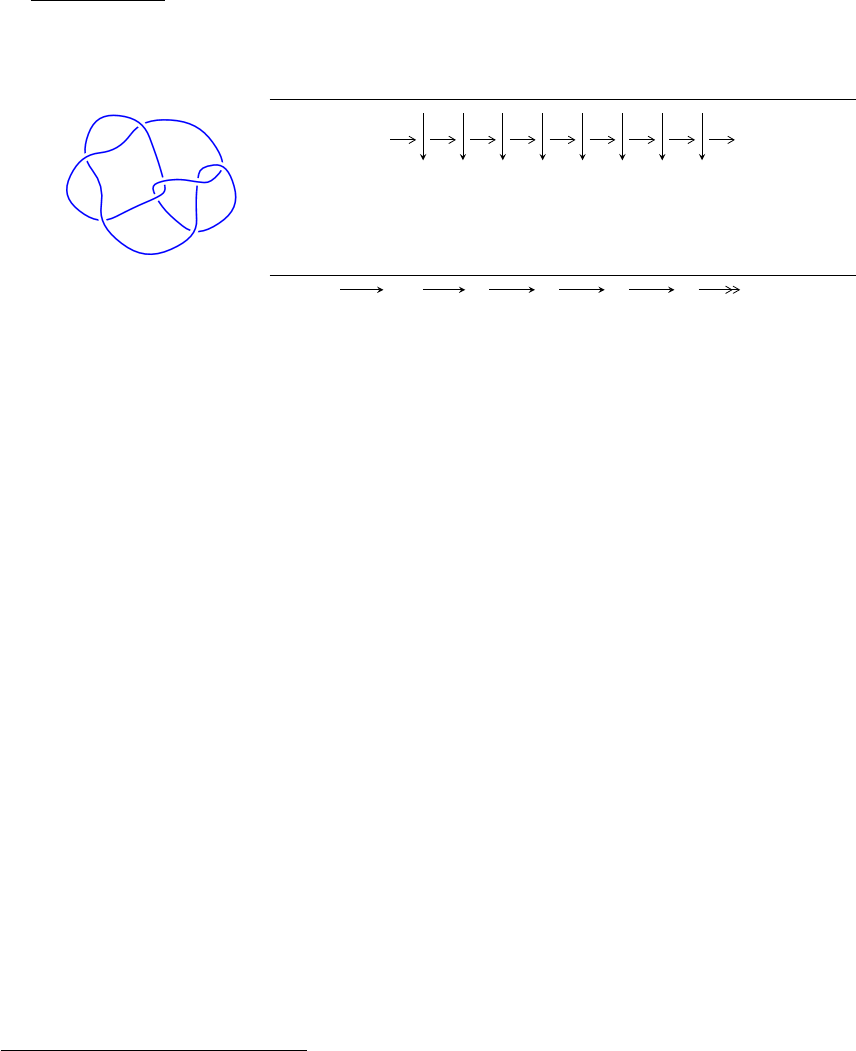8
19
(K8n
3
)
1
Arc Sequences
7 5 7 2 3 1 4 2
Solving Sequence
2,4 5,7
1 3 6 8
c
4
c
1
c
3
c
5
c
8
c
2
, c
6
, c
7
Representation Ideals
I =
2
\
i=1
I
u
i
I
u
1
= hu 1, a, b + 1i
I
u
2
= hu
2
+ 2u 1, b + u, a u 1i
There are 2 irreducible components with 3 representations.
1
The knot diagram image is adapter from “C. Livingston and A. H. Moore, KnotInfo: Table of Knot
Invariants, http://www.indiana.edu/ knotinfo”
1
I. I
u
1
= hu 1, a, b + 1i
(i) Arc colorings
a
2
=
1
0
a
4
=
0
1
a
5
=
1
1
a
7
=
0
1
a
1
=
1
1
a
3
=
0
1
a
6
=
1
0
a
8
=
0
1
(ii) Obstruction class = 1
(iii) Cusp Shapes = 12
2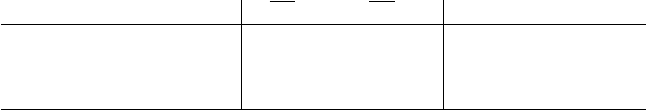(iv) Complex Volumes and Cusp Shapes
Solution to I
u
1
1(vol +
1CS) Cusp shape
u = 1.00000
a = 0
b = 1.00000
3.28987 12.0000
3
II. I
u
2
= hu
2
+ 2u 1, b + u, a u 1i
(i) Arc colorings
a
2
=
1
0
a
4
=
0
u
a
5
=
u
u
a
7
=
u + 1
u
a
1
=
u + 2
2u 1
a
3
=
2u
2u + 1
a
6
=
3u 2
4u + 2
a
8
=
u + 1
2u 1
(ii) Obstruction class = 1
(iii) Cusp Shapes = 12
4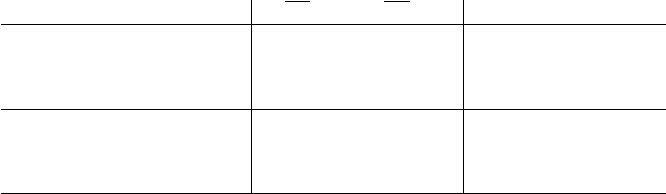(iv) Complex Volumes and Cusp Shapes
Solution to I
u
2
1(vol +
1CS) Cusp shape
u = 2.41421
a = 1.41421
b = 2.41421
18.9167 12.0000
u = 0.414214
a = 1.41421
b = 0.414214
0.822467 12.0000
5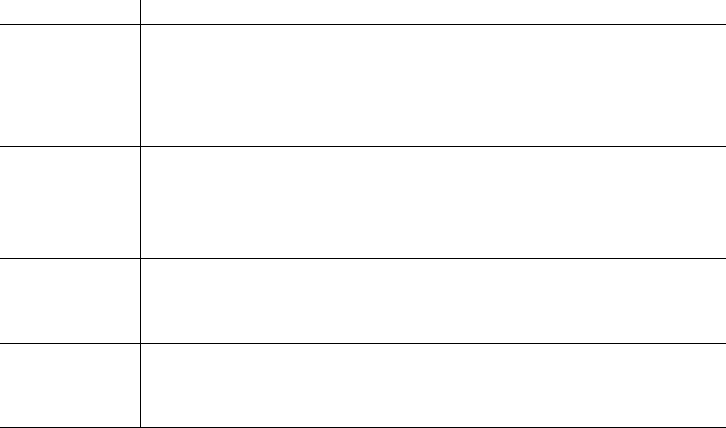III. u-Polynomials
Crossings u-Polynomials at each crossings
c
1
, c
2
, c
4
c
5
(u 1)(u
2
+ 2u 1)
c
3
, c
7
u(u
2
+ 4u + 2)
c
6
(u + 1)(u
2
+ 2u 1)
c
8
(u + 1)(u
2
+ 6u + 1)
6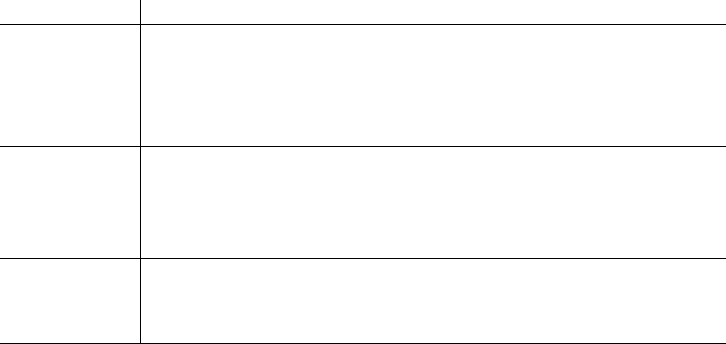IV. Riley Polynomials
Crossings Riley Polynomials at each crossings
c
1
, c
2
, c
4
c
5
, c
6
(y 1)(y
2
6y + 1)
c
3
, c
7
y(y
2
12y + 4)
c
8
(y 1)(y
2
34y + 1)
7